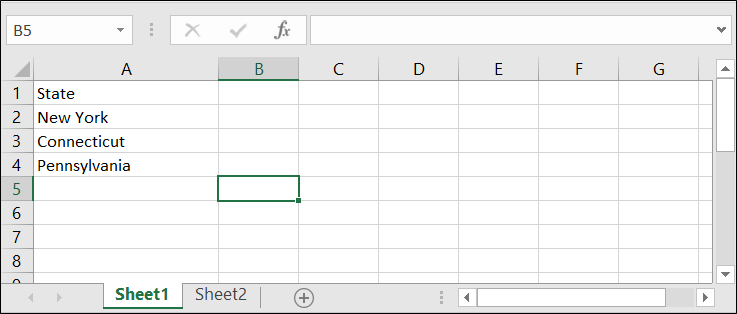Search

# Compare Excel spreadsheets for differences

### The code is set to review sheets named, 'Sheet1' and 'Sheet2'.### Run the code in the second module, and it will create a new workbook which will show the cells containing different text, with an arrow showing the change from Sheet1 to Sheet2.Sub CompareWorksheets(ws1 As Worksheet, ws2 As Worksheet)

Dim r As Long, c As Integer

Dim lr1 As Long, lr2 As Long, lc1 As Integer, lc2 As Integer

Dim maxR As Long, maxC As Integer, cf1 As String, cf2 As String

Dim rptWB As Workbook, DiffCount As Long

Application.ScreenUpdating = False

Application.StatusBar = "Creating the report..."

While Worksheets.Count > 1

Worksheets(2).Delete

Wend

With ws1.UsedRange

lr1 = .Rows.Count

lc1 = .Columns.Count

End With

With ws2.UsedRange

lr2 = .Rows.Count

lc2 = .Columns.Count

End With

maxR = lr1

maxC = lc1

If maxR < lr2 Then maxR = lr2

If maxC < lc2 Then maxC = lc2

DiffCount = 0

For c = 1 To maxC

Application.StatusBar = "Comparing cells " & Format(c / maxC, "0 %") & "..."

For r = 1 To maxR

cf1 = ""

cf2 = ""

On Error Resume Next

cf1 = ws1.Cells(r, c).FormulaLocal

cf2 = ws2.Cells(r, c).FormulaLocal

On Error GoTo 0

If cf1 <> cf2 Then

DiffCount = DiffCount + 1

Cells(r, c).Formula = "'" & cf1 & " <> " & cf2

End If

Next r

Next c

Application.StatusBar = "Formatting the report..."

With Range(Cells(1, 1), Cells(maxR, maxC))

.Interior.ColorIndex = 19

With .Borders(xlEdgeTop)

.LineStyle = xlContinuous

.Weight = xlHairline

End With

With .Borders(xlEdgeRight)

.LineStyle = xlContinuous

.Weight = xlHairline

End With

With .Borders(xlEdgeLeft)

.LineStyle = xlContinuous

.Weight = xlHairline

End With

With .Borders(xlEdgeBottom)

.LineStyle = xlContinuous

.Weight = xlHairline

End With

On Error Resume Next

With .Borders(xlInsideHorizontal)

.LineStyle = xlContinuous

.Weight = xlHairline

End With

With .Borders(xlInsideVertical)

.LineStyle = xlContinuous

.Weight = xlHairline

End With

On Error GoTo 0

End With

Columns("A:IV").ColumnWidth = 20

rptWB.Saved = True

If DiffCount = 0 Then

rptWB.Close False

End If

Set rptWB = Nothing

Application.StatusBar = False

Application.ScreenUpdating = True

MsgBox DiffCount & " cells contain different formulas!", vbInformation, _

"Compare " & ws1.Name & " with " & ws2.Name

End Sub

Sub TestCompareWorksheets()

' compare two different worksheets in the active workbook

CompareWorksheets Worksheets("Sheet1"), Worksheets("Sheet2")

' compare two different worksheets in two different workbooks

CompareWorksheets ActiveWorkbook.Worksheets("Sheet1"), _

Workbooks("WorkBookName.xls").Worksheets("Sheet2")

End Sub